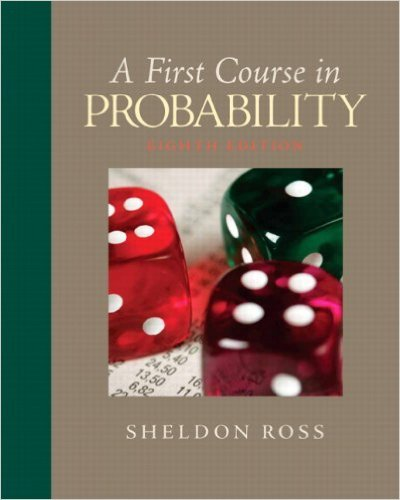> > > Chapter 6

# Solutions for Chapter 6: First Course in Probability 8th Edition## Full solutions for First Course in Probability | 8th Edition

ISBN: 9780136033134Solutions for Chapter 6

Solutions for Chapter 6
4 5 0 256 Reviews
10
4
##### ISBN: 9780136033134

This textbook survival guide was created for the textbook: First Course in Probability, edition: 8. First Course in Probability was written by Sieva Kozinsky and is associated to the ISBN: 9780136033134. Since 61 problems in chapter 6 have been answered, more than 3253 students have viewed full step-by-step solutions from this chapter. This expansive textbook survival guide covers the following chapters and their solutions. Chapter 6 includes 61 full step-by-step solutions.

Key Statistics Terms and definitions covered in this textbook
• 2 k p - factorial experiment

A fractional factorial experiment with k factors tested in a 2 ? p fraction with all factors tested at only two levels (settings) each

• `-error (or `-risk)

In hypothesis testing, an error incurred by rejecting a null hypothesis when it is actually true (also called a type I error).

A formula used to determine the probability of the union of two (or more) events from the probabilities of the events and their intersection(s).

• Analytic study

A study in which a sample from a population is used to make inference to a future population. Stability needs to be assumed. See Enumerative study

• Bernoulli trials

Sequences of independent trials with only two outcomes, generally called “success” and “failure,” in which the probability of success remains constant.

• Biased estimator

Unbiased estimator.

• C chart

An attribute control chart that plots the total number of defects per unit in a subgroup. Similar to a defects-per-unit or U chart.

• Conidence coeficient

The probability 1?a associated with a conidence interval expressing the probability that the stated interval will contain the true parameter value.

• Conidence interval

If it is possible to write a probability statement of the form PL U ( ) ? ? ? ? = ?1 where L and U are functions of only the sample data and ? is a parameter, then the interval between L and U is called a conidence interval (or a 100 1( )% ? ? conidence interval). The interpretation is that a statement that the parameter ? lies in this interval will be true 100 1( )% ? ? of the times that such a statement is made

• Control limits

See Control chart.

• Correction factor

A term used for the quantity ( / )( ) 1 1 2 n xi i n ? = that is subtracted from xi i n 2 ? =1 to give the corrected sum of squares deined as (/ ) ( ) 1 1 2 n xx i x i n ? = i ? . The correction factor can also be written as nx 2 .

• Correlation coeficient

A dimensionless measure of the linear association between two variables, usually lying in the interval from ?1 to +1, with zero indicating the absence of correlation (but not necessarily the independence of the two variables).

• Degrees of freedom.

The number of independent comparisons that can be made among the elements of a sample. The term is analogous to the number of degrees of freedom for an object in a dynamic system, which is the number of independent coordinates required to determine the motion of the object.

• Distribution free method(s)

Any method of inference (hypothesis testing or conidence interval construction) that does not depend on the form of the underlying distribution of the observations. Sometimes called nonparametric method(s).

• Distribution function

Another name for a cumulative distribution function.

• Error of estimation

The difference between an estimated value and the true value.

• Error variance

The variance of an error term or component in a model.

• Expected value

The expected value of a random variable X is its long-term average or mean value. In the continuous case, the expected value of X is E X xf x dx ( ) = ?? ( ) ? ? where f ( ) x is the density function of the random variable X.

• Fractional factorial experiment

A type of factorial experiment in which not all possible treatment combinations are run. This is usually done to reduce the size of an experiment with several factors.

• Goodness of fit

In general, the agreement of a set of observed values and a set of theoretical values that depend on some hypothesis. The term is often used in itting a theoretical distribution to a set of observations.

×

I don't want to reset my password

Need help? Contact support

Need an Account? Is not associated with an account
We're here to help